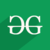GFG App
Open AppBrowser
Continue

# Wand resize() function in Python

Resize image refers to change dimensions of original image in order to convert original image in dimensions that are perfect to use.Scaling Down refers to decrease dimensions of images and make image size smaller. While Scaling Up refers to increase dimensions of an image, and making image size larger.
resize() function is used in order to Resize an image.

Syntax:

`wand.image.resize(width=None, height=None, filter='undefined', blur=1)`

Parameters :

Example 1 :
Input Image :## Python3

 `# import Image from wand.image ` `from` `wand.image ``import` `Image ` ` `  `# read image using Image() function ` `with Image(filename ``=` `'gog.png'``) as img: ` `    ``# resize image using resize() function ` `    ``img.resize(``50``, ``50``, ``filter` `=` `'undefined, blur ``=` `1``) ` ` `  `    ``# save resized image ` `    ``img.save(filename ``=` `'resized_gog.png'``) `

Output :Example 2 :
Input Image :
Input will be from a url.GeeksforGeeks

## Python3

 `# import required libraries ` `import` `urllib3 ` `from` `cStringIO ``import` `StringIO ` `from` `wand.image ``import` `Image ` `from` `wand.display ``import` `display ` ` `  `# load image from url ` `http ``=` `urllib3.PoolManager() ` `r ``=` `http.request(``'GET'``, ``'https://media.geeksforgeeks.org/wp-content/uploads/geeksforgeeks-6.png'``) ` `f ``=` `StringIO(r.data) ` ` `  `# read image using Image() function ` `with Image(``file``=``f) as img: ` ` `  `    ``# resize image using resize() function ` `    ``img.resize(``400``, ``300``) ` ` `  `    ``# save image  ` `    ``img.save(filename ``=` `'gogurl.png'``) ` ` `  `    ``# display final image ` `    ``display(img) `

Output :My Personal Notes arrow_drop_up